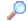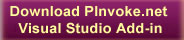SearchDirectory

Desktop Functions:

Smart Device Functions:GlossaryShow Recent ChangesSubscribe (RSS)Misc. Pages
SOCKET_OPTION_NAME (ws2_32)

Summary
Enumerates all possible options used with getsockopt and setsockopt.

#### C# Signature:

public enum SOCKET_OPTION_NAME : int
{
#region IPPROTO_IP Socket Options

/// <summary>
/// Set/get IP options.
/// </summary>
IP_OPTIONS          =   1,

/// <summary>
/// Header is included with data.
/// </summary>
IP_HDRINCL          =   2,

/// <summary>
/// IP type of service.
/// </summary>
IP_TOS              =   3,

/// <summary>
/// IP TTL (hop limit).
/// </summary>
IP_TTL              =   4,

/// <summary>
/// IP multicast interface.
/// </summary>
IP_MULTICAST_IF         =   9,

/// <summary>
/// IP multicast TTL (hop limit).
/// </summary>
IP_MULTICAST_TTL        =   10,

/// <summary>
/// IP multicast loopback.
/// </summary>
IP_MULTICAST_LOOP       =   11,

/// <summary>
/// Add an IP group membership.
/// </summary>

/// <summary>
/// Drop an IP group membership.
/// </summary>
IP_DROP_MEMBERSHIP      =   13,

/// <summary>
/// Don't fragment IP datagrams.
/// </summary>
IP_DONTFRAGMENT         =   14,

/// <summary>
/// Join IP group/source.
/// </summary>

/// <summary>
/// Leave IP group/source.
/// </summary>
IP_DROP_SOURCE_MEMBERSHIP   =   16,

/// <summary>
/// Block IP group/source.
/// </summary>
IP_BLOCK_SOURCE         =   17,

/// <summary>
/// Unblock IP group/source.
/// </summary>
IP_UNBLOCK_SOURCE       =   18,

/// <summary>
/// Receive packet information.
/// </summary>
IP_PKTINFO          =   19,

/// <summary>
/// Receive packet hop limit.
/// </summary>
IP_HOPLIMIT         =   21,

/// <summary>
/// Allow/block broadcast reception.
/// </summary>

/// <summary>
/// Receive arrival interface.
/// </summary>
IP_RECVIF           =   24,

/// <summary>
/// </summary>

/// <summary>
/// Enable/Disable an interface list.
/// </summary>
IP_IFLIST           =   28,

/// <summary>
/// Add an interface list entry.
/// </summary>

/// <summary>
/// Delete an interface list entry.
/// </summary>
IP_DEL_IFLIST           =   30,

/// <summary>
/// IP unicast interface.
/// </summary>
IP_UNICAST_IF           =   31,

/// <summary>
/// Set/get IPv6 routing header.
/// </summary>
IP_RTHDR            =   32,

/// <summary>
/// </summary>
IP_RECVRTHDR        =   38,

/// <summary>
/// Packet traffic class.
/// </summary>
IP_TCLASS           =   39,

/// <summary>
/// Receive packet traffic class.
/// </summary>
IP_RECVTCLASS           =   40,

/// <summary>
/// Original Arrival Interface Index.
/// </summary>
IP_ORIGINAL_ARRIVAL_IF      =   47,

/// <summary>
/// Receive ECN codepoints in the IP header
/// </summary>
IP_ECN              =   50,

/// <summary>
/// Receive extended packet information.
/// </summary>
IP_PKTINFO_EX           =   51,

/// <summary>
/// WFP's Connection Redirect Records
/// </summary>
IP_WFP_REDIRECT_RECORDS     =   60,

/// <summary>
/// WFP's Connection Redirect Context
/// </summary>
IP_WFP_REDIRECT_CONTEXT     =   70,

#endregion

#region IPPROTO_IPV6 Socket Options

/// <summary>
/// Set/get IPv6 hop-by-hop options.
/// </summary>
IPV6_HOPOPTS        =   1,

/// <summary>
/// Header is included with data.
/// </summary>
IPV6_HDRINCL        =   2,

/// <summary>
/// IP unicast hop limit.
/// </summary>
IPV6_UNICAST_HOPS       =   4,

/// <summary>
/// IP multicast interface.
/// </summary>
IPV6_MULTICAST_IF       =   9,

/// <summary>
/// IP multicast hop limit.
/// </summary>
IPV6_MULTICAST_HOPS     =   10,

/// <summary>
/// IP multicast loopback.
/// </summary>
IPV6_MULTICAST_LOOP     =   11,

/// <summary>
/// Add an IP group membership.
/// </summary>

/// <summary>
///
/// </summary>

/// <summary>
/// Drop an IP group membership.
/// </summary>
IPV6_DROP_MEMBERSHIP    =   13,

/// <summary>
/// Drop an IP group membership.
/// </summary>
IPV6_LEAVE_GROUP        =   IPV6_DROP_MEMBERSHIP,

/// <summary>
/// Don't fragment IP datagrams.
/// </summary>
IPV6_DONTFRAG           =   14,

/// <summary>
/// Receive packet information.
/// </summary>
IPV6_PKTINFO        =   19,

/// <summary>
/// Receive packet hop limit.
/// </summary>
IPV6_HOPLIMIT           =   21,

/// <summary>
/// Set/get IPv6 protection level.
/// </summary>
IPV6_PROTECTION_LEVEL       =   23,

/// <summary>
/// Receive arrival interface.
/// </summary>
IPV6_RECVIF         =   24,

/// <summary>
/// </summary>

/// <summary>
/// Offset to checksum for raw IP socket send.
/// </summary>
IPV6_CHECKSUM           =   26,

/// <summary>
/// Treat wildcard bind as AF_INET6-only.
/// </summary>
IPV6_V6ONLY         =   27,

/// <summary>
/// Enable/Disable an interface list.
/// </summary>
IPV6_IFLIST         =   28,

/// <summary>
/// Add an interface list entry.
/// </summary>

/// <summary>
/// Delete an interface list entry.
/// </summary>
IPV6_DEL_IFLIST         =   30,

/// <summary>
///  IP unicast interface.
/// </summary>
IPV6_UNICAST_IF         =   31,

/// <summary>
/// Set/get IPv6 routing header.
/// </summary>
IPV6_RTHDR          =   32,

/// <summary>
/// </summary>
IPV6_RECVRTHDR          =   38,

/// <summary>
/// Packet traffic class.
/// </summary>
IPV6_TCLASS         =   39,

/// <summary>
/// Receive packet traffic class.
/// </summary>
IPV6_RECVTCLASS         =   40,

/// <summary>
/// Receive ECN codepoints in the IP header.
/// </summary>
IPV6_ECN            =   50,

/// <summary>
/// Receive extended packet information.
/// </summary>
IPV6_PKTINFO_EX         =   51,

/// <summary>
/// WFP's Connection Redirect Records
/// </summary>
IPV6_WFP_REDIRECT_RECORDS   =   60,

/// <summary>
/// WFP's Connection Redirect Context
/// </summary>
IPV6_WFP_REDIRECT_CONTEXT   =   70,

#endregion

#region SOL_SOCKET Socket Options

/// <summary>
/// configuration info for service provider
/// </summary>
PVD_CONFIG          =   0x3001,

/// <summary>
/// turn on debugging info recording
/// </summary>
SO_DEBUG            =   0x0001,

/// <summary>
/// socket has had listen()
/// </summary>
SO_ACCEPTCONN           =   0x0002,

/// <summary>
/// allow local address reuse
/// </summary>

/// <summary>
/// keep connections alive
/// </summary>
SO_KEEPALIVE        =   0x0008,

/// <summary>
/// just use interface addresses
/// </summary>
SO_DONTROUTE        =   0x0010,

/// <summary>
/// permit sending of broadcast msgs
/// </summary>

/// <summary>
/// bypass hardware when possible
/// </summary>
SO_USELOOPBACK          =   0x0040,

/// <summary>
/// linger on close if data present
/// </summary>
SO_LINGER           =   0x0080,

/// <summary>
/// leave received OOB data in line
/// </summary>
SO_OOBINLINE        =   0x0100,

/// <summary>
///
/// </summary>
SO_DONTLINGER           =   ~SO_LINGER,

/// <summary>
/// disallow local address reuse
/// </summary>

/// <summary>
/// send buffer size
/// </summary>
SO_SNDBUF           =   0x1001,

/// <summary>
/// receive buffer size
/// </summary>
SO_RCVBUF           =   0x1002,

/// <summary>
/// send low-water mark
/// </summary>
SO_SNDLOWAT         =   0x1003,

/// <summary>
/// receive low-water mark
/// </summary>
SO_RCVLOWAT         =   0x1004,

/// <summary>
/// send timeout
/// </summary>
SO_SNDTIMEO         =   0x1005,

/// <summary>
/// </summary>
SO_RCVTIMEO         =   0x1006,

/// <summary>
/// get error status and clear
/// </summary>
SO_ERROR            =   0x1007,

/// <summary>
/// get socket type
/// </summary>
SO_TYPE             =   0x1008,

/// <summary>
/// get socket 5-tuple state
/// </summary>
SO_BSP_STATE        =   0x1009,

/// <summary>
/// ID of a socket group
/// </summary>
SO_GROUP_ID         =   0x2001,

/// <summary>
/// the relative priority within a group
/// </summary>
SO_GROUP_PRIORITY       =   0x2002,

/// <summary>
/// maximum message size
/// </summary>
SO_MAX_MSG_SIZE         =   0x2003,

/// <summary>
/// WSAPROTOCOL_INFOA structure
/// </summary>
SO_PROTOCOL_INFOA       =   0x2004,

/// <summary>
/// WSAPROTOCOL_INFOW structure
/// </summary>
SO_PROTOCOL_INFOW       =   0x2005,

/// <summary>
///enable true conditional accept:
// connection is not ack-ed to the
// other side until conditional
// function returns CF_ACCEPT
/// </summary>
SO_CONDITIONAL_ACCEPT       =   0x3002,

/// <summary>
/// pause accepting new connections
/// </summary>
SO_PAUSE_ACCEPT         =   0x3003,

/// <summary>
/// get/set the compartment for a socket
/// </summary>
SO_COMPARTMENT_ID       =   0x3004,

/// <summary>
/// enable port scalability
/// </summary>
SO_PORT_SCALABILITY     =   0x3006,

SO_CONNDATA         =   0x7000,
SO_CONNOPT          =   0x7001,
SO_DISCDATA         =   0x7002,
SO_DISCOPT          =   0x7003,
SO_CONNDATALEN          =   0x7004,
SO_CONNOPTLEN           =   0x7005,
SO_DISCDATALEN          =   0x7006,
SO_DISCOPTLEN           =   0x7007,

/// <summary>
/// Option for opening sockets for synchronous access.
/// </summary>
SO_OPENTYPE         =   0x7008,
SO_MAXDG            =   0x7009,
SO_MAXPATHDG        =   0x700A,
SO_UPDATE_ACCEPT_CONTEXT    =   0x700B,
SO_CONNECT_TIME         =   0x700C,

#endregion

#region IPPROTO_TCP Socket Options

/// <summary>
/// Enables or disables the Nagle algorithm for TCP sockets. This option is disabled (set to FALSE) by default.
/// </summary>
TCP_NODELAY         =   0x0001,

/// <summary>
/// If TRUE, the service provider implements the expedited data as specified in RFC-1222. Otherwise, the Berkeley Software Distribution
/// (BSD) style (default) is used. This option can be set on the connection only once. Once this option is set on, this option cannot
/// be turned off. This option is not required to be implemented by service providers.
/// </summary>
TCP_EXPEDITED_1122          =   0x0002,

/// <summary>
/// Enables or disables the Nagle algorithm for TCP sockets. This option is disabled (set to FALSE) by default.
/// </summary>
TCP_BSDURGENT           =   0x7000,

#endregion

#region IPPROTO_UDP Socket Options

/// <summary>
/// When TRUE, UDP datagrams are sent with the checksum of zero. Required for service providers. If a service provider does not have a mechanism
/// to disable UDP checksum calculation, it may simply store this option without taking any action. This option is not supported for IPv6.
/// </summary>
UDP_NOCHECKSUM          =   1,

/// <summary>
/// Set/get UDP-Lite checksum coverage
/// </summary>
UDP_CHECKSUM_COVERAGE       =   20

#endregion
}

None.

#### Alternative Managed API:

Do you know one? Please contribute it!

None.

#### Sample Code:

Documentation

Do you have...

• helpful tips or sample code to share for using this API in managed code?
• corrections to the existing content?
• variations of the signature you want to share?
• additional languages you want to include?

Select "Edit This Page" on the right hand toolbar and edit it! Or add new pages containing supporting types needed for this API (structures, delegates, and more).Access PInvoke.net directly from VS:Terms of UseEdit This PageFind ReferencesShow Printable VersionRevisions Show changes